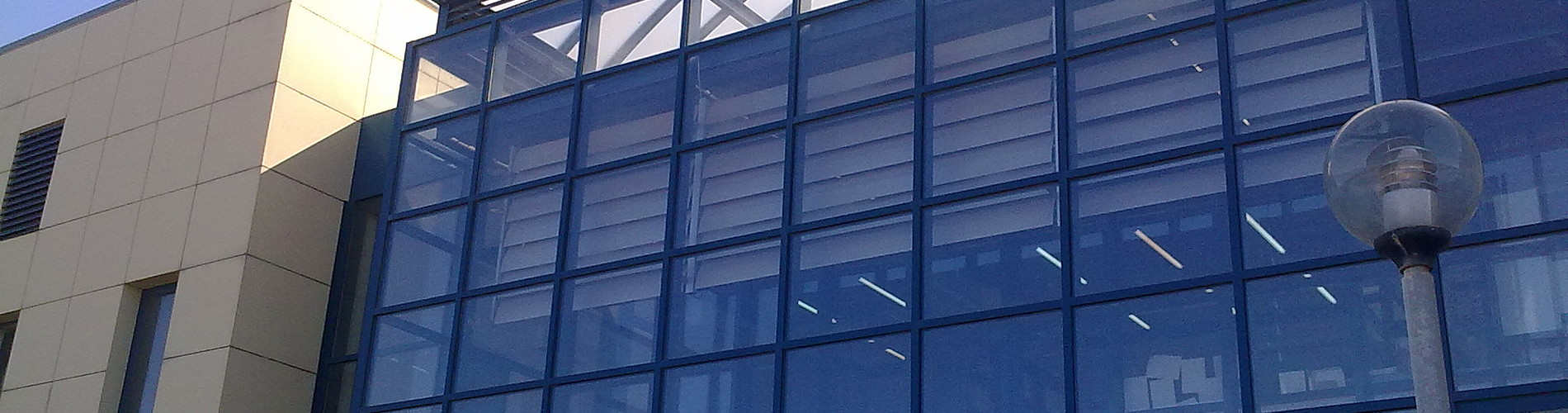School of Chemical and Environmental Engineering

Now offering two distinct diplomas: Chemical Engineering and Environmental Engineering

# Linear Algebra

1. COURSE INFORMATION:

 School Environmental Engineering Course Level Undergraduate Course ID MATH 201 Semester 2nd Course Category Required Course Modules Instruction Hours per Week ECTS Lectures and Laboratory Exercises 5 Th=4, E=0, L=1 6 Course Type General Background Prerequisites Instruction/Exam Language Greek The course is offered to Erasmus students No Course URL https//www.eclass.tuc.gr/courses/MHPER313/  (in Greek)

2. LEARNING OUTCOMES

 Learning Outcomes This course  aims to introduce the basic ideas and techniques of linear algebra for use in many other lecture courses, as well as in solving linear systems that arise from the modeling of natural phenomena. Topics include systems of linear equations and their solutions, matrices and matrix algebra, inverse matrices, determinants; real n-dimensional vector spaces, abstract vector spaces and their axioms, linear transformations; inner products (dot products), orthogonality, cross products, and their geometric applications; subspaces, linear independence, bases for vector spaces, dimension, matrix rank; eigenvectors, eigenvalues, matrix diagonalization. Some applications of linear algebra will be discussed, such as linear regression (least squares), elementary Markov chains.Upon successful completion of this course, the student will be able tounderstand  the basic  vectors and matrix operations and their properties,understand the  notions of linear dependence and independence of vectors, the notion of the basis and  understand spanning set and subspace,solve regular or irregular systems of linear equations  using elimination methods,apply the basic technics to to determine whether a matrix is invertible and compute the inverse if it is,compute the fundamental subspaces of a matrix, (null space,row space, column space) the rank of a matrix and how these notions are related with the solutions of a linear system of equations,compute the determinant, the characteristic polynomial, the eigenvalues and eigenvectors  of a square matrix  and find  its diagonalization if it is,use  the  Gram-Schmidt process  and  orthonomalize the basis of a finite dimensional vector space, find the projection onto a subspace of a finite dimensional space and the complement of  a subspace,understand  the usage  of linear algebra in several applications:best approximation,least squares elementary Markov chains. General Competencies/Skills Review, analyse and synthesise data and information, with the use of necessary technologies) Work autonomouslyWork in teamsWork in an international frame

3. COURSE SYLLABUS

 Contents: Introduction to linear algebra and the algebra of vectors and matrices. Direct methods of solving systems of linear equations. Determinants. Vector spaces, subspaces. Linear dependence, independence, basis of a vector space. Fundamental subspaces of a matrix. Eigenvector,eigenvalues. Diagonalization  and applications. Gram-Schmidt process of orthonormalization, least square method. Iterative methods for solving linear systems.Laboratories:  Introduction  to  MATLAB  software with emphasis on the problems and the theory of Linear Algebra as well as the linear systems solving algorithms. Supported Operations for Vectors and Matrices. Creating, Concatenating  vectors and matrices,expanding matrices. Functions and Subfunctions.

4. INSTRUCTION and LEARNING METHODS - ASSESSMENT

 Lecture Method Direct (face to face) Use of Information and Communication Technology Specialized software, E-class support Instruction Organisation Activity Workload per Semester (hours) - Lectures 52 - Lab assignments 13 - Autonomous study 85 Course Total 150 Assessment Method Ι. Written final examination (80%)ΙΙ. Laboratory exercises  (20%).